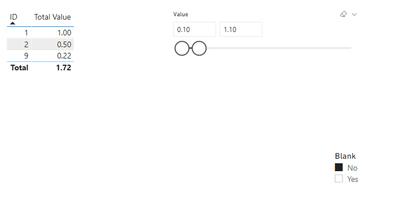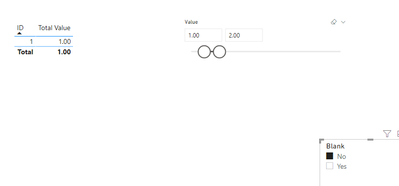cancel
Showing results for
Did you mean:Frequent Visitor

## Measure to use range slicer which can include blanks

Hi,
I would like to use a slicer [tick box] to allow the user to decide if he wants to see only the values from a range slicer or in addition blank values.
I have a dataset with a range of numbers from 0 to 10, quite randomly distributed. There are also blanks in this dataset.
Possible dataset:

 ID Value 1 1,00 2 0,50 3 5,00 4 5 7,50 6 7 10,00 8 9 0,22 10 4,50

Now the user should choose a value range, say [0.1, 1.1] (this will explicitly exclude blanks in PowerBI as I understand/see it)
If the user tickes "No" blanks in the tickbox slicer, the outcome should be:

 ID Value 1 1 2 0,5 9 0,22

And if "Yes" (I want to see blanks) is selected, the outcome should be:

 ID Value 1 1 2 0,5 4 6 8 9 0,22

`I tried to use a measure of the following style:measure = var _min = MINX(ALLSELECTED(Table), Table[Value])var _max = MAXX(ALLSELECTED(Table), Table[Value]) var _blanks = IF(ISFILTERED(BlankFilter[Filter]), max(BlankFilter[Filter]), blank())RETURN IF(_blanks = "Yes", CALCULATE(SUM(Table[Value]), FITLER( ALL(Table), ISBLANK(Table[Value]) || Table[Value] >= _min && Table[Value] <= _max), CALCULATE(SUM(Table`

I encounter the following problems:
1. If I do not apply the filter (value range slicer) to the visual I am looking at (table), the min and max values are not correct
2. If I do apply the filter (value range slicer) to the visual, the blanks are always filtered out but the min and max values are of course correct.

I understand that this is due to the filter contect of the measure. But I thought, with ALL() in the measure, I get rid of the filter or with the ALLSELECTED() I can access the value range slicer?
Thanks
Best regards

1 ACCEPTED SOLUTIONSuper User

Hi @tmul ,

Create a table with the values and another with the slicer:

``SLICER VALUES = FILTER( DISTINCT('Table (2)'[Value]), 'Table (2)'[Value] <> BLANK())``

This table should be used for the slicer of the values.

``````Total Value =
SWITCH (
TRUE (),
SELECTEDVALUE ( 'Show/Blanks'[Blank] ) = "Yes"
&& SUM ( 'FACTTABLE'[Value] ) = BLANK (), "",
SELECTEDVALUE ( 'Show/Blanks'[Blank] ) = "Yes"
&& CALCULATE (
SUM ( 'FACTTABLE'[Value] ),
'FACTTABLE'[Value] IN VALUES ( 'SLICER VALUES'[Value] )
)
<> BLANK (),
CALCULATE (
SUM ( 'FACTTABLE'[Value] ),
'FACTTABLE'[Value] IN VALUES ( 'SLICER VALUES'[Value] )
),
SELECTEDVALUE ( 'Show/Blanks'[Blank] ) = "No",
CALCULATE (
SUM ( 'FACTTABLE'[Value] ),
'FACTTABLE'[Value] IN VALUES ( 'SLICER VALUES'[Value] )
)
)``````

Has you can see below and in the attach file the selection show and hides the blanks:Regards

Miguel Félix

Proud to be a Super User!

Check out my blog: Power BI em Português4 REPLIES 4Super User

Hi @tmul ,

Create a table with the values and another with the slicer:

``SLICER VALUES = FILTER( DISTINCT('Table (2)'[Value]), 'Table (2)'[Value] <> BLANK())``

This table should be used for the slicer of the values.

``````Total Value =
SWITCH (
TRUE (),
SELECTEDVALUE ( 'Show/Blanks'[Blank] ) = "Yes"
&& SUM ( 'FACTTABLE'[Value] ) = BLANK (), "",
SELECTEDVALUE ( 'Show/Blanks'[Blank] ) = "Yes"
&& CALCULATE (
SUM ( 'FACTTABLE'[Value] ),
'FACTTABLE'[Value] IN VALUES ( 'SLICER VALUES'[Value] )
)
<> BLANK (),
CALCULATE (
SUM ( 'FACTTABLE'[Value] ),
'FACTTABLE'[Value] IN VALUES ( 'SLICER VALUES'[Value] )
),
SELECTEDVALUE ( 'Show/Blanks'[Blank] ) = "No",
CALCULATE (
SUM ( 'FACTTABLE'[Value] ),
'FACTTABLE'[Value] IN VALUES ( 'SLICER VALUES'[Value] )
)
)``````

Has you can see below and in the attach file the selection show and hides the blanks:Regards

Miguel Félix

Proud to be a Super User!

Check out my blog: Power BI em PortuguêsFrequent Visitor

Tanks a lot for the help @MFelix ! I could implement it in my report.  Now I am only in front of another rather small issue. The ID does not need to be unique. Then as I see it, the measure would sum up the different values for one ID. Do I need to use the SUMX command instead somehow or do you have another suggestion?
Let's say that ID 1 appears two times, one times with 1 and one times with 0.9. Now the ID 1 would not be shown, but I would like to have it 🙂 The best thing would be if I could get all IDs from it which satisfy the filters for each row. That sounds like a SUMX or?Super User

Hi @tmul ,

This depends on the filtering do you want to compare the SUM of the ID compared to the slicer or the value of each ID.

Has you can see keeping the same filters has previous 0,1 and 1.1 the value that appears is the 1.9However if you change the slicer from 1 to 2 for example it only shows 1:In this case do you want to appear 1 or 1.9 because is within the range.

Regards

Miguel Félix

Proud to be a Super User!

Check out my blog: Power BI em PortuguêsFrequent Visitor

Thanks! I will implement it and come back to you with some feedback. 👍Announcements#### Exclusive opportunity for Women!

Join us for a free, hands-on Microsoft workshop led by women trainers for women where you will learn how to build a Dashboard in a Day!#### Power Platform Conference-Power BI and Fabric Sessions

Join us Oct 1 - 6 in Las Vegas for the Microsoft Power Platform Conference.Top Solution Authors
Top Kudoed Authors
Users online (4,127)